Algebra 2 5-9 Guided Practice: Transforming Polynomial Functions
starstarstarstarstarstarstarstarstarstar
by Matthew Richardson
| 6 Questions1
10
Solve It! The graph of the parent cubic function f(x) = is shown in blue. The red graph is a transformation g of the parent function f. What is an equation for g?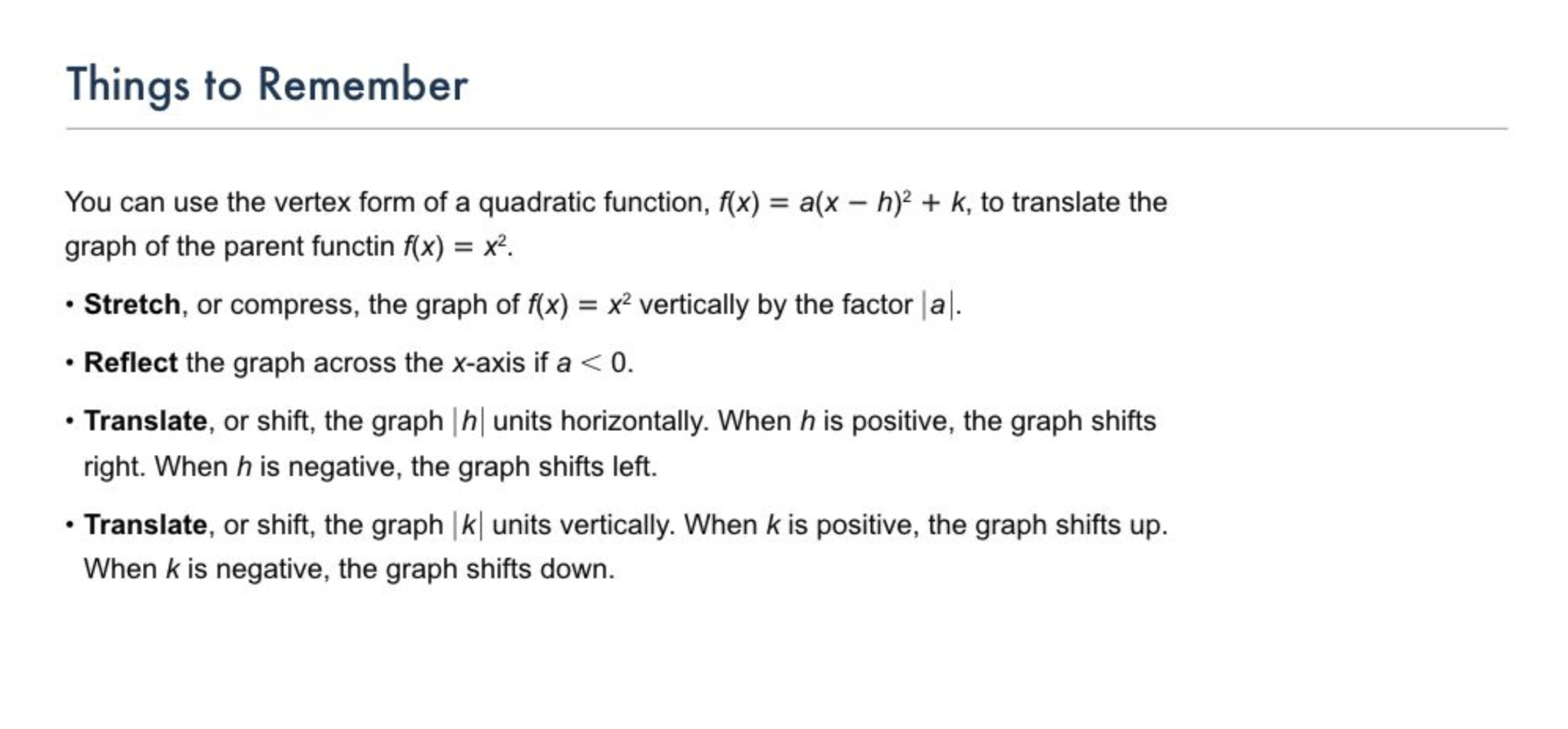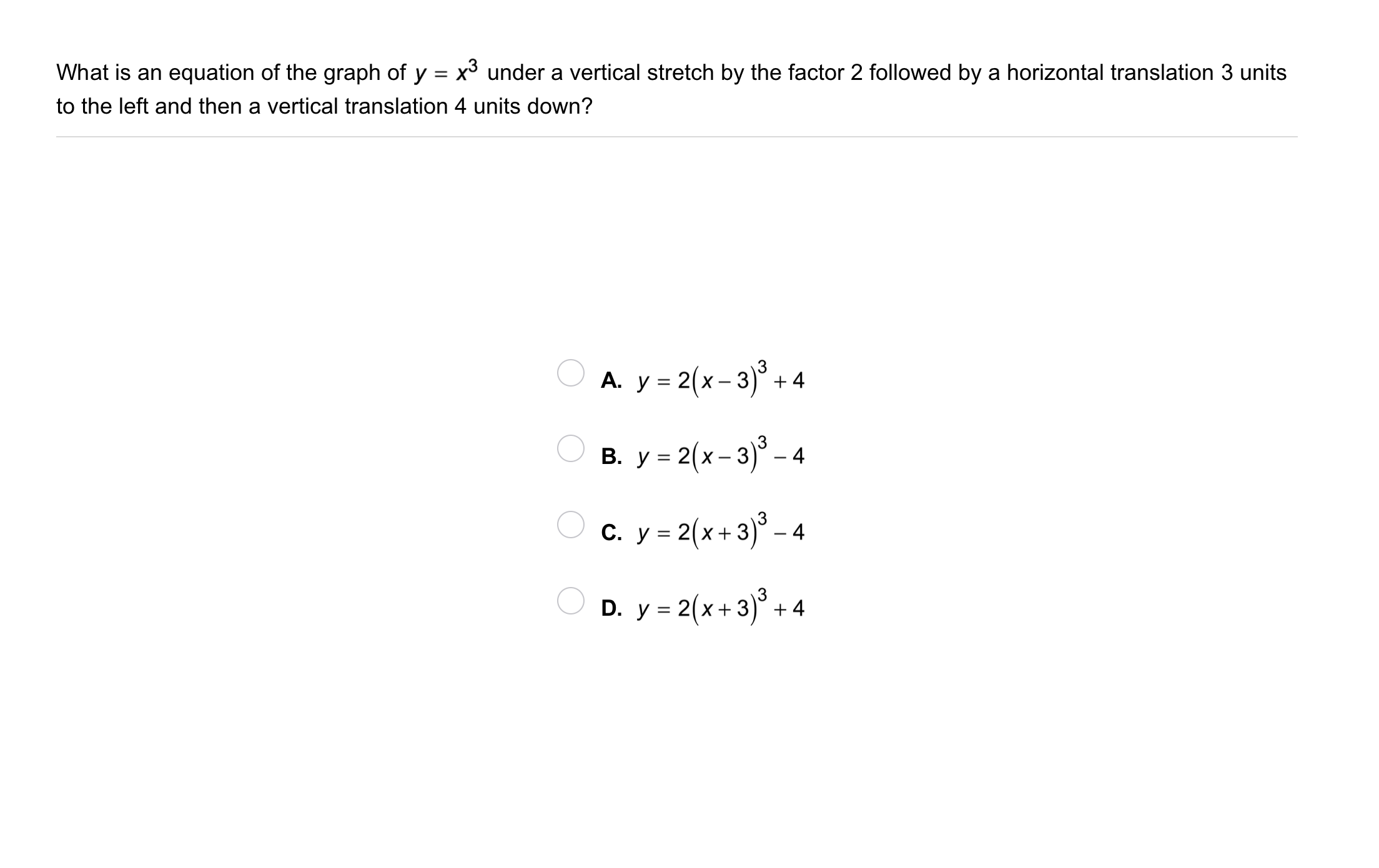2
2
10
Problem 1 Got It?
A
B
C
D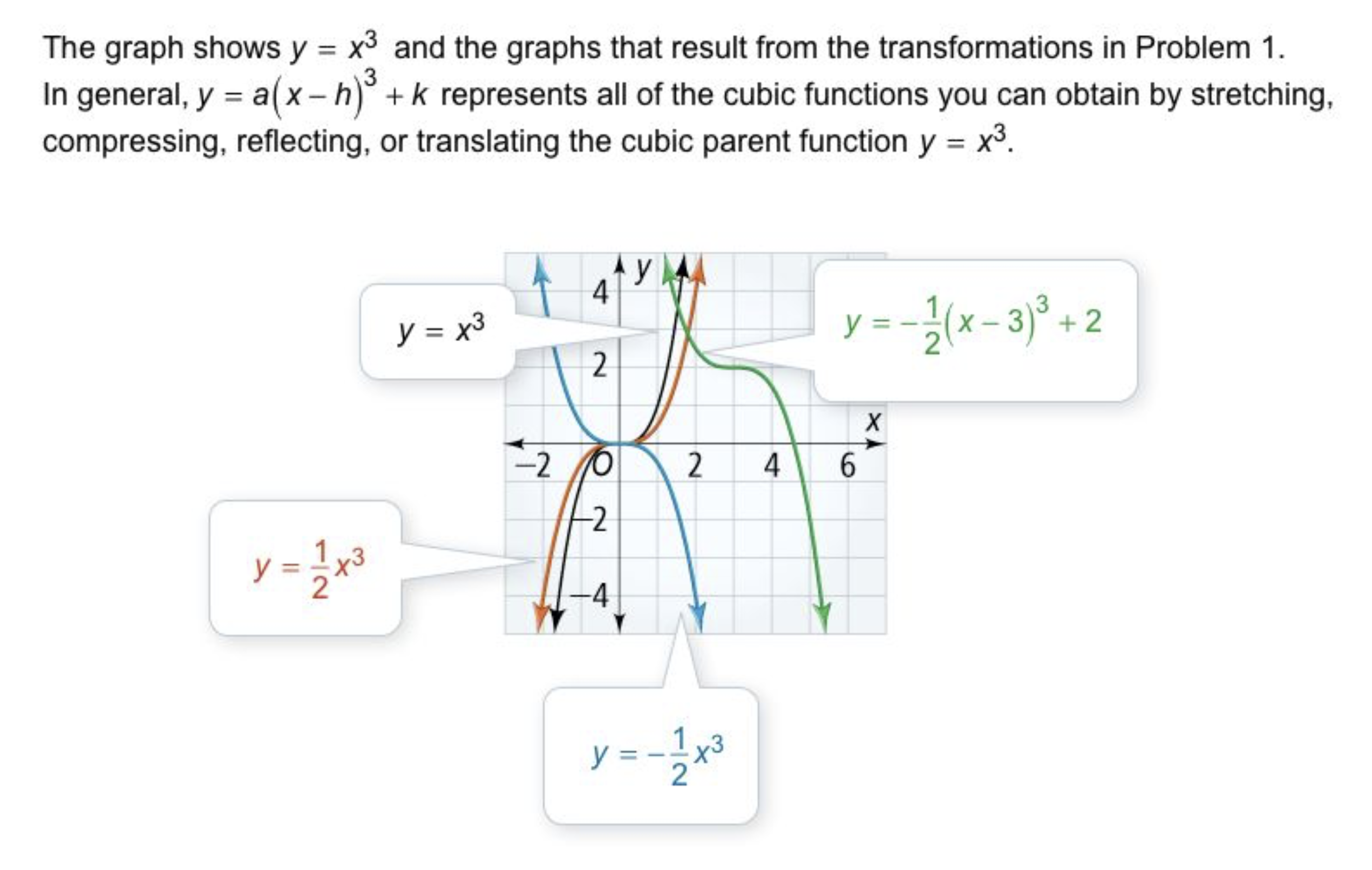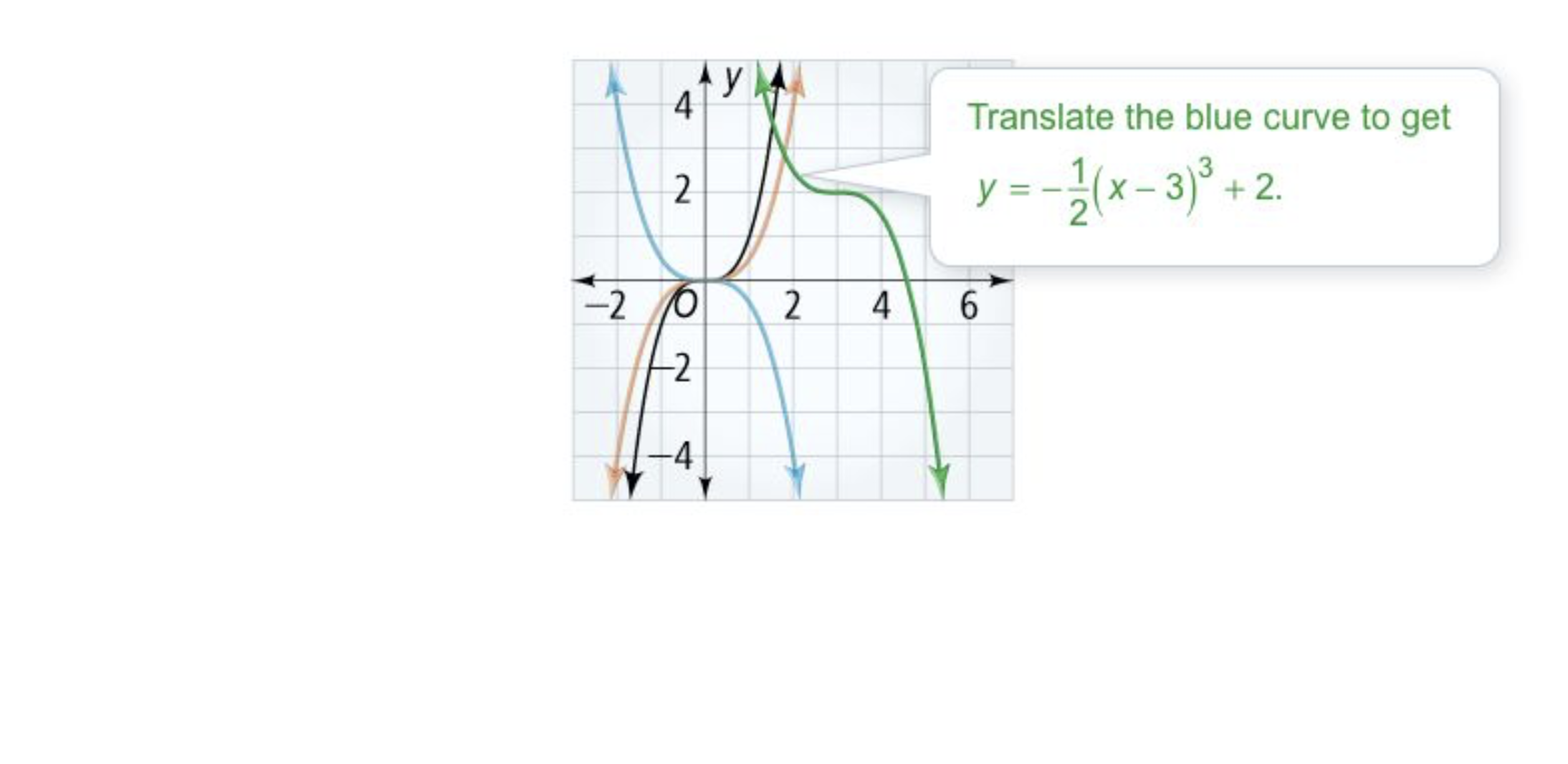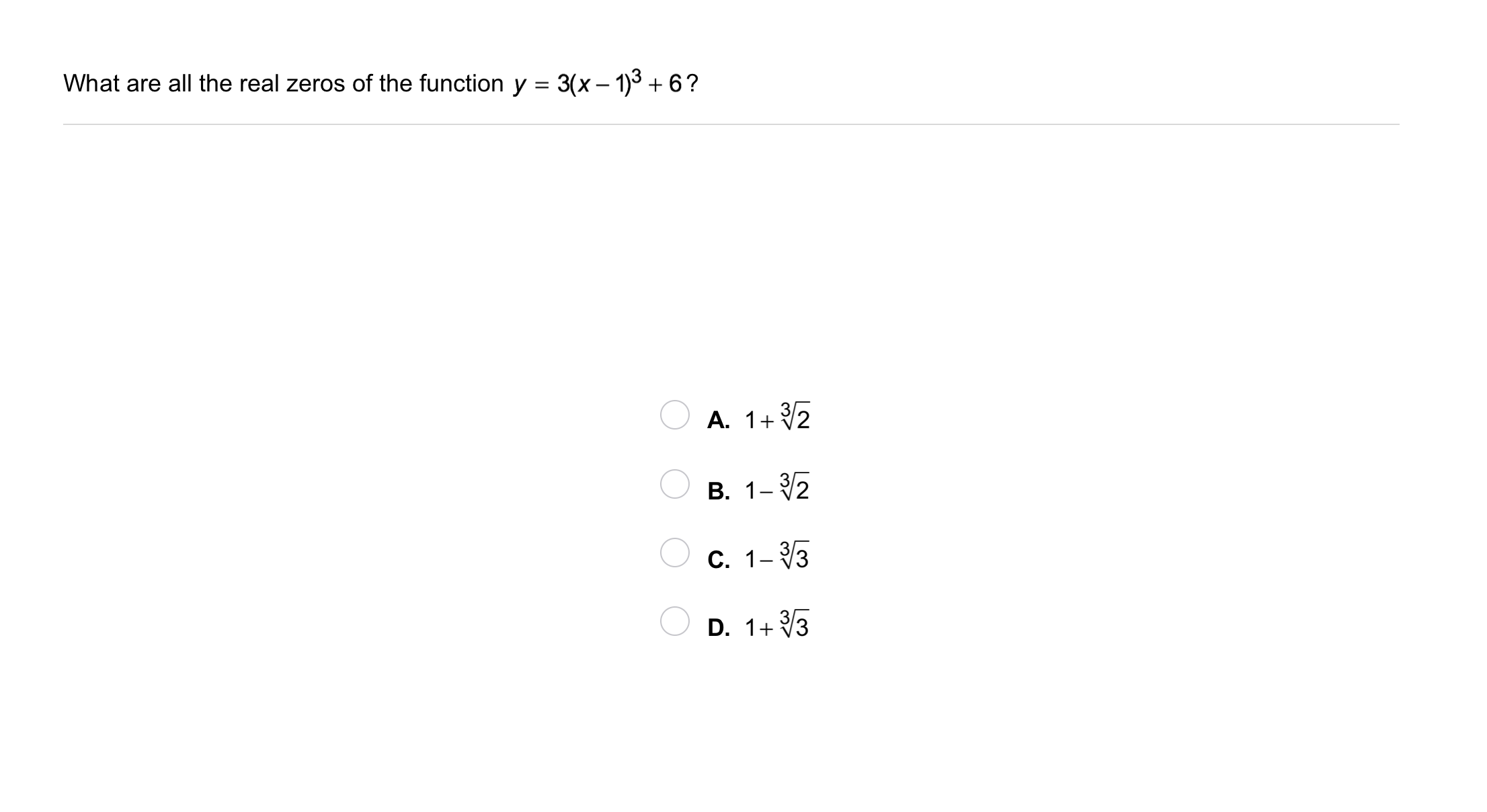3
3
10
Problem 2 Got It?
A
B
C
D4
10
Problem 3 Got It? What is a quartic function f(x) with only two real zeros, x = 0 and x = 6?

5
10
Problem 3 Got It? Reasoning: Does -f(x) have the same zeros? Explain.
Yes; -f(x) is f(x) reflected across the x-axis, so the zeros will stay the same.
Yes; -f(x) is f(x) reflected across the y-axis, so the zeros will stay the same.
No; -f(x) is f(x) reflected across the x-axis, so the zeros will not stay the same.
No; -f(x) is f(x) reflected across the y-axis, so the zeros will not stay the same.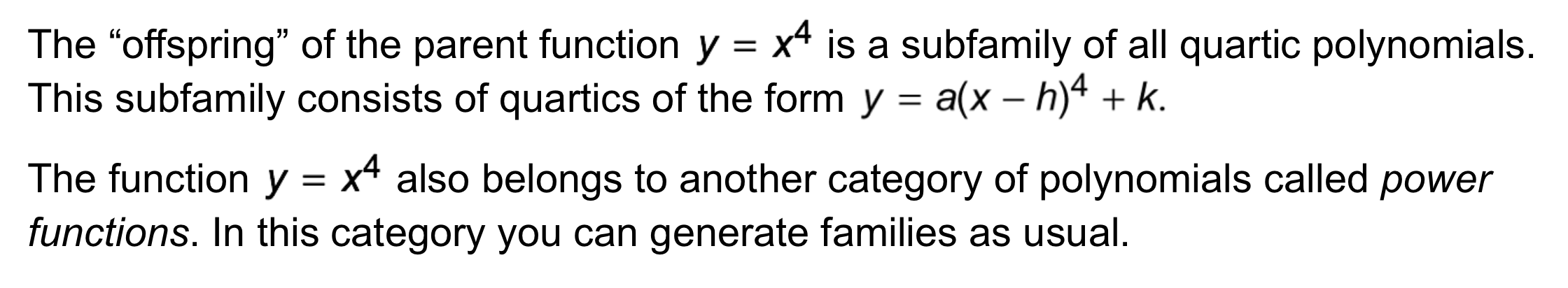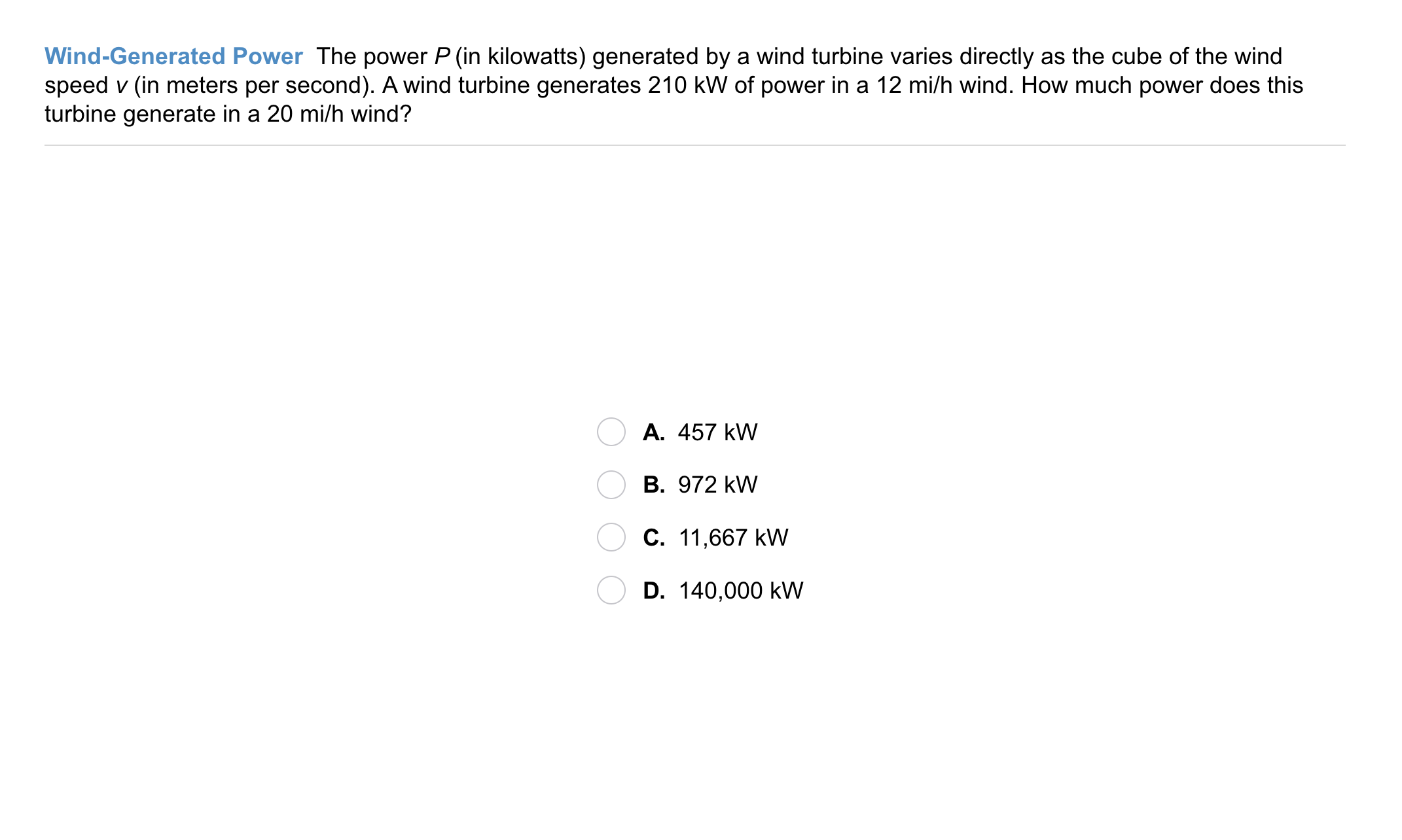6
6
10
Problem 4 Got It? Wind-Generated Power: The power P (in kilowatts) generated by a wind turbine varies directly as the cube of the wind speed v (in meters per second). A wind turbine generates 210 kW of power in a 12 mi/h wind. How much does this turbine generate in a 20 mi/h wind?
A
B
C
D